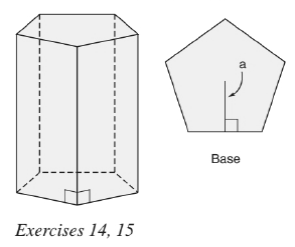Chapter 9.1, Problem 15E### Elementary Geometry for College St...

6th Edition
Daniel C. Alexander + 1 other
ISBN: 9781285195698

#### Solutions

Chapter
Section### Elementary Geometry for College St...

6th Edition
Daniel C. Alexander + 1 other
ISBN: 9781285195698
Textbook Problem
31 views

# In the regular pentagonal prism shown above, suppose that each base edge measures 9.2 cm and that the apothem of the base measures 6.3 cm. The altitude of the prism measures 14.6 cm.a) Find the lateral area of the prism.b) Find the total area of the prism.c) Find the volume of the prism.To determine

a)

To find:

The lateral area of the prism.

Explanation

Approach:

The lateral area of any solid is the area of all the number of lateral sides that it have, excluding the area of the top and the bottom of the solid.

The lateral area (L) of any solid prison with the perimeter of the base of the prism as (P) and altitude ‘h’ is given by the formula

L=Ph.

Here, P is the perimeter of any one of the base of the prism and which is given by the sum of all the sides of the base.

Calculation:

Given,

The measure of the base edge of the pentagonal prism = 9.2 cm.

The measure of the apothem of the base of the prism = 6.3 cm.

The altitude of the prism = 14.6 cm.

The lateral area of any prism of altitude ‘h’ and of base perimeter P is given by

L=hP

Here, we have

h = 14

To determine

b)

To find:

The total area of the prism.

To determine

c)

To find:

The volume of the prism.

### Still sussing out bartleby?

Check out a sample textbook solution.

See a sample solution

#### The Solution to Your Study Problems

Bartleby provides explanations to thousands of textbook problems written by our experts, many with advanced degrees!

Get Started

#### Find more solutions based on key concepts